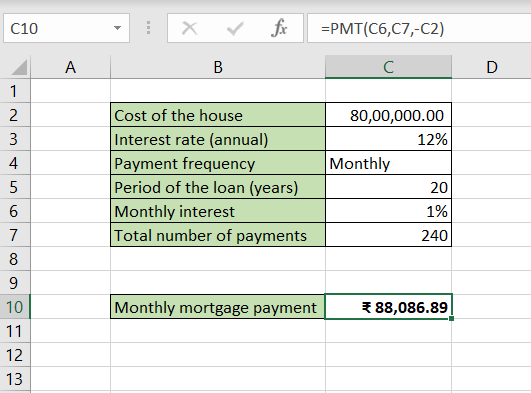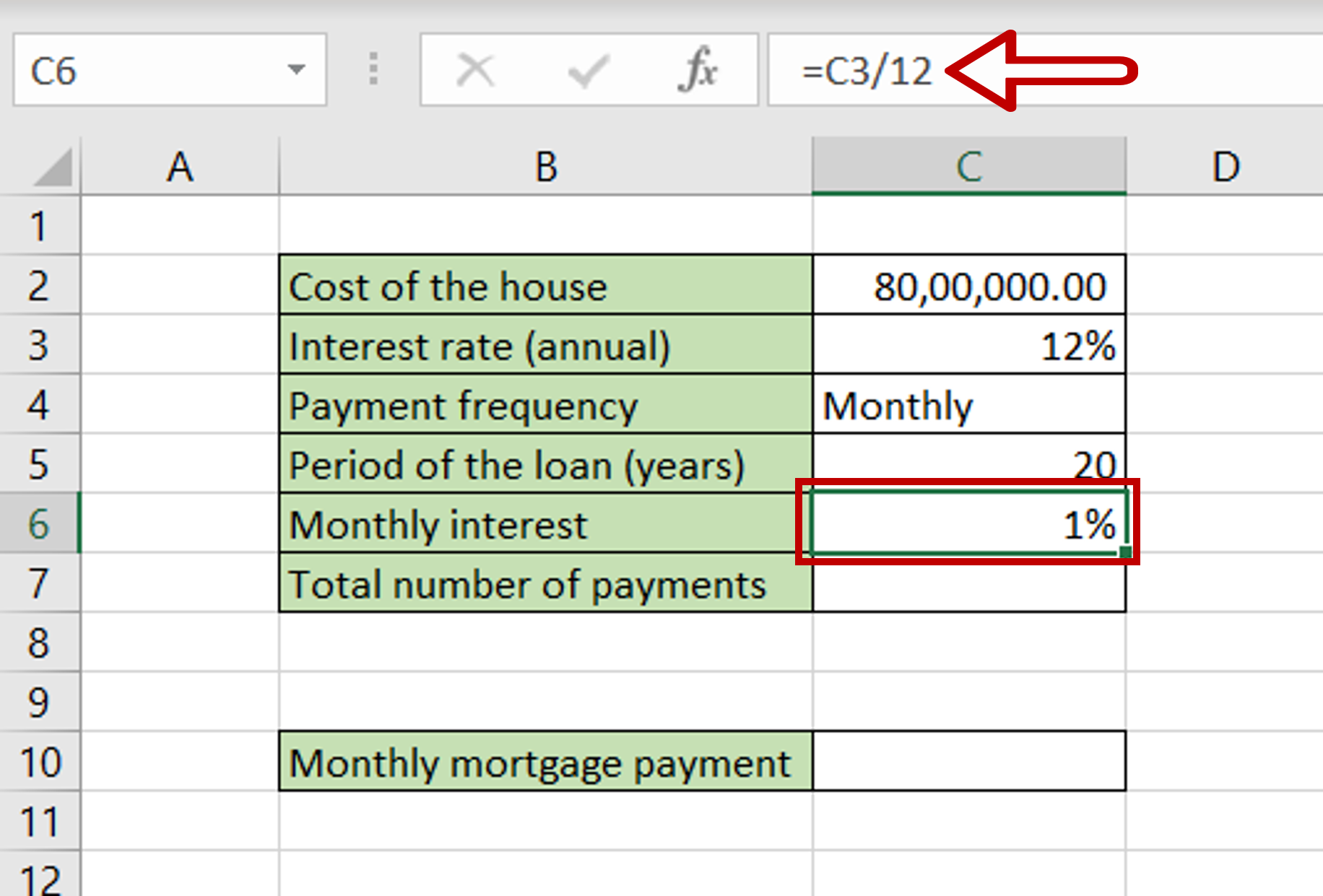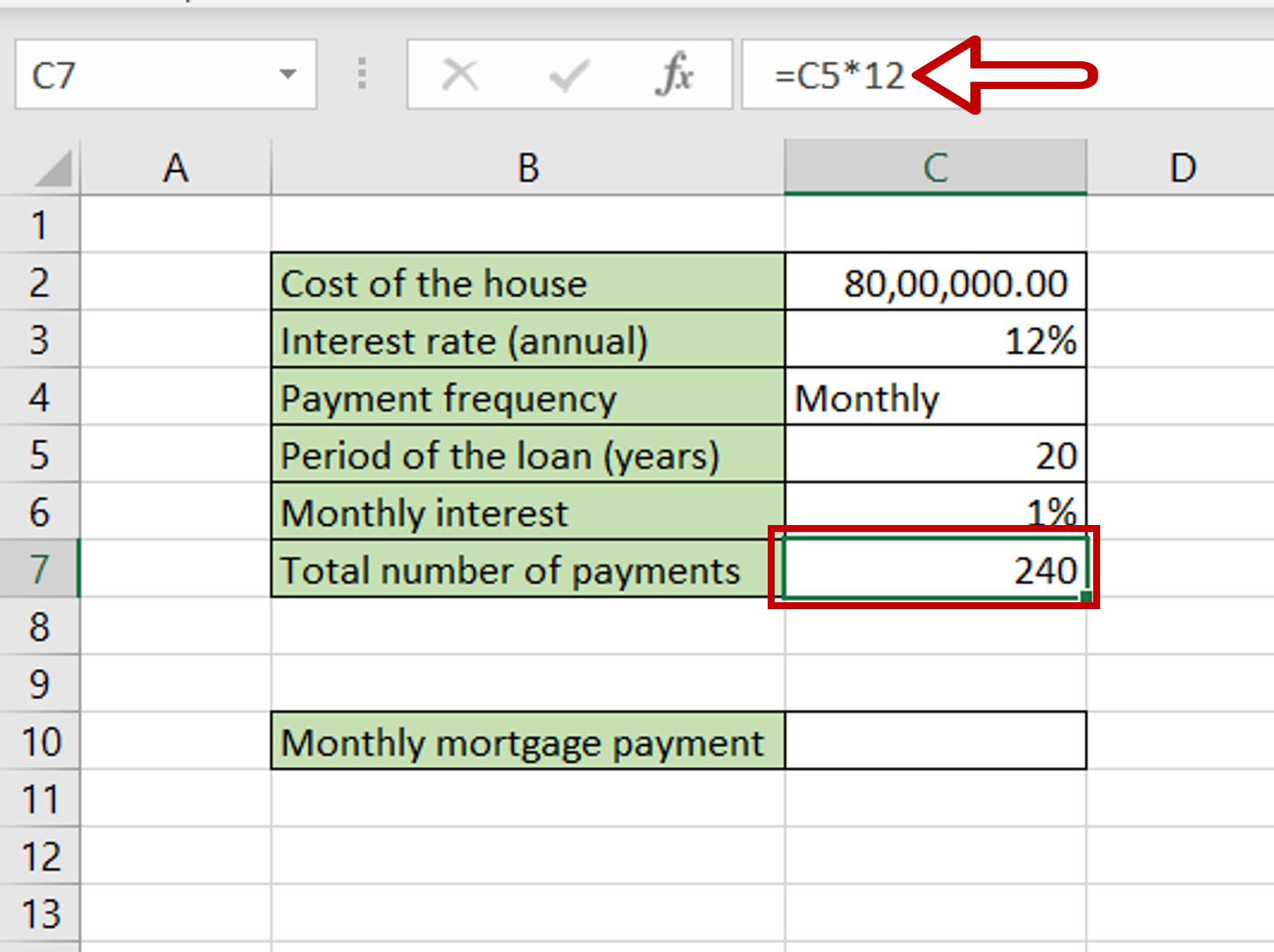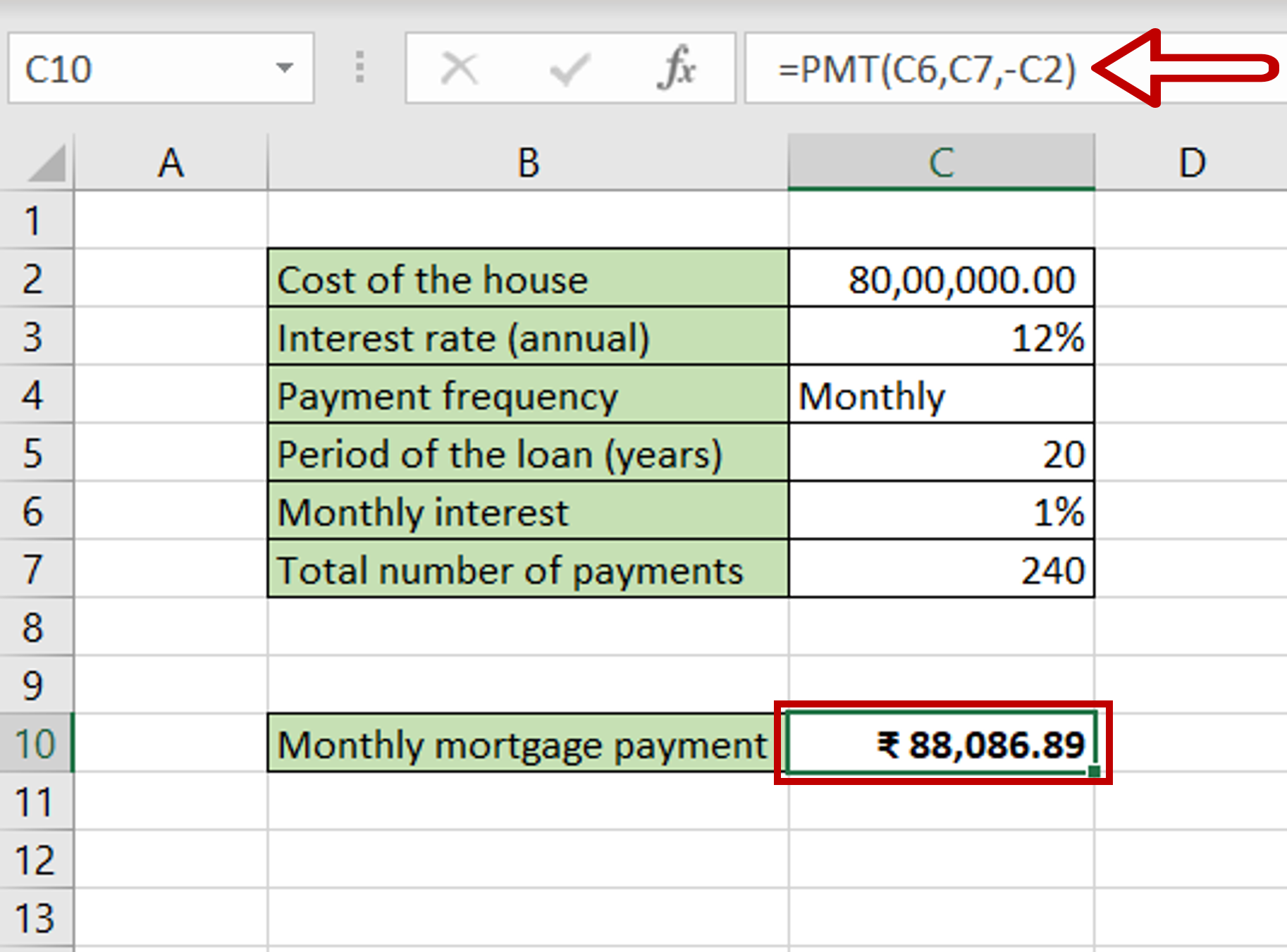# How to calculate monthly mortgage payments in Excel

You can watch a video tutorial here.

For example, you can build a calculator to calculate the monthly mortgage payment based on the cost of a house. Using this, you can compare houses of various costs to understand which mortgage payment would be the most feasible for you. In Excel, you can use the PMT function to compute the monthly mortgage payment.

1. PMT() function: this calculates the monthly payment for a mortgage
1. Syntax: PMT(rate, nper, pv, [fv], [type])
1. rate: the interest rate
2. nper: the total number of payments
3. pv: the principal
4. [fv] optional: remaining cash balance after the last payment is made, by default this is zero
5. [type] optional: specify whether the payment is due at the beginning or end of the loan

In this example, given the basic parameters of the mortgage, we will compute the inputs for the PMT() function using mathematical operations.Excel is frequently used for calculations and supports all basic mathematical operations. Using formulas, you can use Excel to build different types of calculators in which you only need to change the values to get the result. This saves you the problem of doing each step of the calculation repeatedly.

### Step 1 – Find the monthly interest rate– Select the cell for the monthly interest to be displayed
– Type the formula using cell references:
= Interest rate (annual)/12
– Press Enter

### Step 2 – Find the total number of payments– Select the cell for the total number of payments to be displayed
– Type the formula using cell references:
= Period of the loan (years) * 12
– Because the payment frequency is monthly, the number is multiplied by 12
– Press Enter

### Step 3 – Create the formula using PMT()– Select the cell for the monthly mortgage payment to be displayed
– Type the formula using cell references:
= PMT(Monthly interest, Total number of payments, – Cost of the house)
Note: The PMT() function returns a negative number, so a minus sign is put in front of the ‘Cost of the house’ to make the result a positive number.
– Press Enter